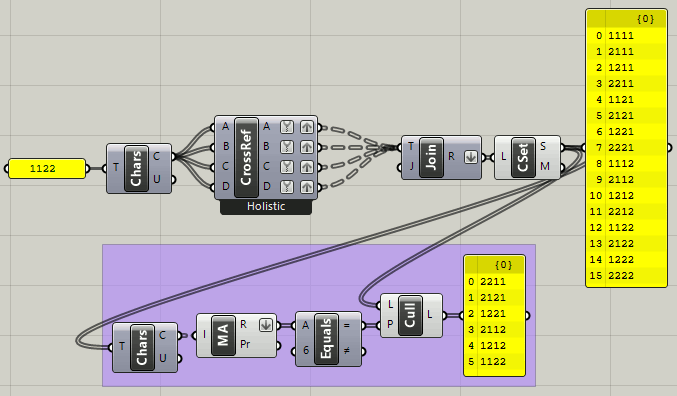# Partition Numbers

Hey there. Got a question, hope you can help me. :') So I want to partition a Number ‘X’(is this the right word lol?), lets say 6 into 4 new numbers, for example a: 1, b: 1, c: 3, d:1, the sum would be six again oc. Any ideas? Furthermore I want each of this new partitial numbers to have an individual maximum. I added a screenshot and a gh-file, hope it helps understanding.
So if c:3 is over the individual max, I need to reduce that number to 2 in that case, but at the same time add the difference to one of the other numbers, while not getting over that other individual max.
The sum of the individual max is ofc the maximum for my ‘X’, id fix that with an if expression.
Any ideas? Would be a huge help. Really need that code :S

PartitionNumber.gh (14.5 KB)

Hello,

So to rephrase and simplify you would like to partition an integer , applying a maximum but no minimum to the individual values so with a sum of 6 and a max of 2 the only solutions are:

222
2211
21111
111111

Is that right? This is called the partition sum problem, I think

Would you be happy with a solution using a Python or C# component?

Alternatively you need to set each cell in turn to the minimum of:

• the cell maximum or
• the partition sum(6) less the sum filled so far less the number of remaining unfilled cells (3, 2, 1,0)

Here implemented in Python:

``````n = 4 # number of cels
summ = 6
maxis = [2,]*n
for i, _ in enumerate( answers):

Thanks for your time. Python is fine oc.Not exactly I’m afraid. There always should be 4 output numbers. But the maximum of each is individual. So for example, without cap the possibiltys for the integer 6 are
1122
1311
1410
0600
2022
and so on, BUT
I want a maximum for each to be defined, so for example
2122 is the maximum
so the only possibiltys now would be
2121
1122
2112
2022
Sum always 6, but first number not higher then 2, second not higher then 1, 3rd not higher then 2, same for 4th. Does that sound logical? Sorry if not :S

Ok so my python solution above should give one solution for an given set of input conditions.
Do you need every solution, including ‘anagrams ‘ ?

Oke, Gotta admit, I am not sure, how to implement this correctly.
Mhm. I want the solution to be randomiced, so that I get 1 of this solutions with a random seed output.
No anagrams, if I understand it right. The order of the maximums will always be the same, the maximums should me parametric tho, so in another case it could be a max of 4322. Got 4 inputs for that from another code.
I’ll need to research on how to implement python scripts first, tho, still a beginner :S

If done in python, i’d need something like this as result. I don’t have much experience with scripting tho :S

And what is the maximum sum and maximum number of cells? With a sum of 10 a brute force approach is fine. A sum of 20 to 30 requires some caching of sub-solutions to get an answer in a reasonable number of seconds.

This kind of works but it is more likely to give large values for the first cells !!!

``````from random import randint

n = 4 # number of cels
summ =  x
maxis = [a,b,c,d]

for i, _ in enumerate( answers):
minval = 1
print(i, minval, maxval)

outa, outb, outc, outd = answers``````

This sounds like combinations and permutations? CrossRef might be useful?
But I’m not into the weeds with you guys on this one, sorry. I don’t understand the question.

P.S. Quicky variation of David Rutten’s model (first link above):permutations_2019Nov19a.gh (13.2 KB)

Looks very promising, but if I copy this to the python print, it tells me: Runtime error (UnboundNameException): name ‘randint’ is not defined

Traceback:
line 9, in script

Any clue, why or what to do?

This is great, thanks. The input 1122 is the only thing, I am curious about. Its a simple text input, sum is 6 (perfect), but as soon, as I change the sum to 5 (X), i’d need to create a new textfield. So the question is, how can I get 4 random numbers, with a sum of X.

Did you include the first line `from random import randint` ?

What version of rhino are you using? I am on 6.18 for Windows. I know there have been some problems with the random module in other versions of Rhino

Oh, my bad, sry. Ok, its somehow working, BUT it seems, that with a value of 6 for x, the sum is always x-1. Furthermore, the individual maxis aren’t correct. I got a 1211 as solution, but the maxis are 2122.

Again, I’m not really following this and don’t really understand the question. Using only integers?

One more distracted hack:

permutations_2019Nov19b.gh (18.4 KB)

I did it. Thank you really much for your timePartitionNumber_solved_2019_Nov20.gh (16.4 KB)